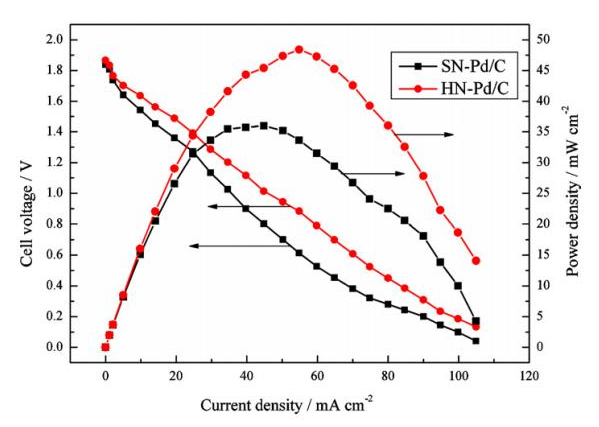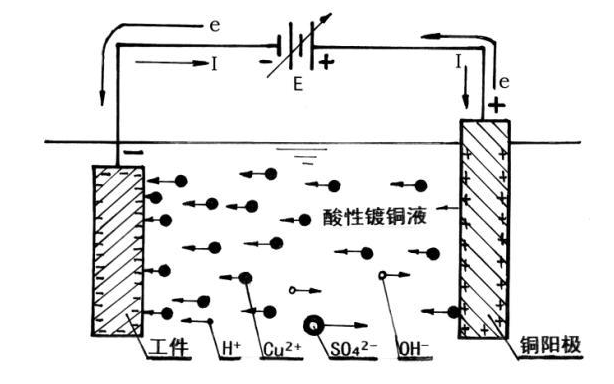# Electrode polarization

Polarization refers to the phenomenon where things are polarized under certain conditions, causing their properties to deviate from their original state. Of course, electrode polarization occurs between the two electrodes (that is, the positive and negative electrodes, or the anode and cathode) of the electrolytic cell or battery. Let the positive charge accumulate around one electrode or restore the ability to continuously lose electrons; at the same time let the negative charge accumulate around the other electrode or restore the ability to continue accepting electrons.

contents

• 1. The concept of electrode polarization
• 2. Reasons for electrode polarization
• 3. The result of electrode polarization

## 1. The concept of electrode polarization

Under irreversible conditions, when a current flows through the electrode, an irreversible electrode reaction occurs. At this time, the electrode potential is different from the reversible electrode potential. The phenomenon that the electrode potential and the reversible electrode potential deviate when the electrode has a current is called electrode polarization. The characteristics of electrode polarization are: the cathode potential is more negative than the equilibrium potential (cathode polarization), and the anode potential is more positive than the equilibrium potential (anode polarization).

In the case of a reversible battery, the entire battery is in an electrochemical equilibrium state, and the two electrodes are also in equilibrium respectively. The electrode potential is determined by the Nernst equation, which is the balanced electrode potential. At this time, the current through the electrode is zero, that is, the rate of electrode reaction is zero. If a non-zero current is passed through the electrode, the electrode potential must deviate from the value of the equilibrium electrode potential. This phenomenon is called electrode polarization.

Electrode polarization (electrode polarization) When the Electronic conductor is in contact with the solution in the surrounding rock, it will form a galvanic double layer, resulting in a potential jump. This potential jump is called the electrode potential when the electronic conductor is in contact with the solution. When there is an external electric field, the relatively balanced electrode potential value will change. Usually the difference between the electrode potential under the action of constant current density and the relatively balanced electrode potential is called electrode polarization. Common ones are electrochemical polarization, concentration polarization and so ON. The electromotive force caused by electrode polarization is called overvoltage.## 2. Reasons for electrode polarization

The reason for electrode polarization: when there is an external electric field, the relatively balanced electrode potential value will change, leading to the appearance of electrode polarization.

1. When there is an external electric field, the relatively balanced electrode potential value will change. Generally, the deviation of the electrode potential under a certain current density from the relatively balanced electrode potential is called electrode polarization. Common ones are electrochemical polarization (activation polarization), concentration polarization and so on. The electromotive force caused by electrode polarization is called overpotential (overvoltage).

2. Electrode polarization can be divided into concentration polarization and chemical polarization
When the current passes through the battery or electrolytic cell, if the entire electrode process is controlled by the diffusion and convection of the electrolyte, the electrolyte concentration near the two poles is different from the body of the solution, causing the electrode potential of the anode and cathode to deviate from the equilibrium electrode potential. This phenomenon is called “concentration polarization”. It can be eliminated by vigorously stirring the solution. Chemical polarization is related to the activation energy of the reaction and cannot be eliminated.## 3. The result of electrode polarization

An electrode, in the case of reversibility, has a certain degree of electrification on the electrode, and establishes the corresponding electrode potential jr. When a current flows through the electrode, if the electrode reaction at the electrode-solution interface does not proceed fast enough, resulting in a change in the degree of charge of the electrode, the electrode potential can also deviate from jr. Take the electrode (Pt)H2(g)|H as an example. When the reduction effect of the cathode occurs, since the rate of H changing to H2 is not fast enough, the electrons reaching the cathode cannot be consumed in time when the current passes, making the electrode more reversible. In this case, there is more negative electricity, so that the electrode potential becomes lower than jr. This lower potential can promote the activation of the reactant, that is, accelerate the conversion of H into H2. When (Pt)H2(g)|H is used as an anode to oxidize, because the rate of H2 changing to H is not fast enough, the lack of electrons on the electrode due to the current passing through is more serious than in the reversible situation, resulting in the electrode with More positive charge, so the electrode potential becomes higher than jr. This higher potential is conducive to promoting the activation of the reactants and accelerating the transformation of H2 into H. Extending this to all electrodes, a conclusion of universal significance can be obtained: when there is a current passing through, due to the slowness of the electrochemical reaction, the degree of charging of the electrode is different from that in the reversible situation, which leads to the phenomenon that the electrode potential deviates from jr, which is called “Activation polarization” or “electrochemical polarization”. When the electrode is activated and polarized, as in the concentration polarization, the cathode potential always becomes lower than jr, and the anode potential always becomes higher than jr. The absolute value of the difference between the electrode potential jI and jr caused by activation polarization is called “activation overpotential”. The magnitude of the activation overpotential is a measure of the activation polarization of the electrode.